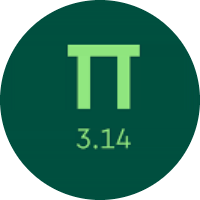# Learning the Units of Measurement

In this quiz, you will learn about different units of measurement. You will learn about comparing and converting the customary units of length, weight, volume, etc. You will also get to know about the metric units.

Start Quiz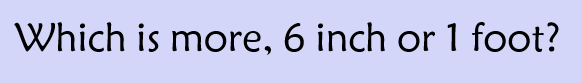6 inch

1 foot

Neither, both are equal

Find the value of X and Y.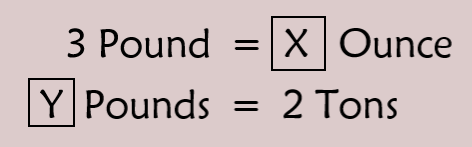X = 48, Y = 4000

X = 48, Y = 2000

X = 24, Y = 4000

X = 32, Y = 3000

Find the value of m and n.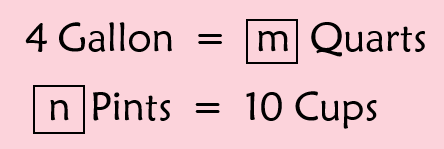m = 18, n =5

m = 20, n = 10

m = 16, n = 5

m = 24, n = 5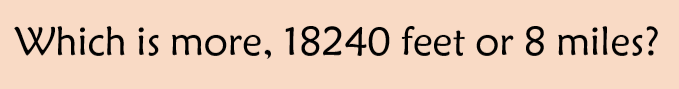18240 feet

8 miles

Convert.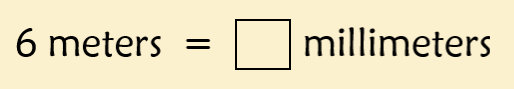600

6000

60

Convert.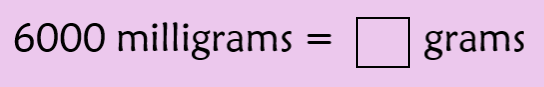60

600

64 liters

4000 liters

Neither; both are equal

Find.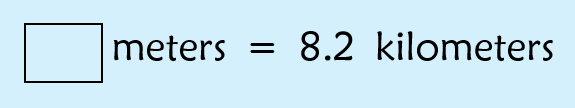820

8200

82

Convert.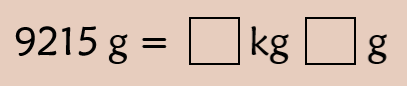92 kg 15 g

9 kg 215 g

9.2 kg 150 g

9 g 215 kg

Quiz/Test Summary
Title: Learning the Units of Measurement
Questions: 9
Contributed by: